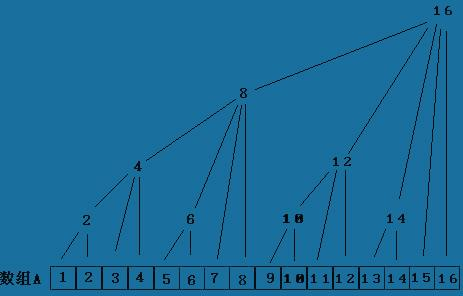# acronix

posts - 64, comments - 4, trackbacks - 0, articles - 0

首先这里我不具体解释 int Lowbit（） { i + （- i ) }  来源，我只想强调的是数组C【i】保存的是C[i - 2^k +1] 到C[i] 区间的状态，k 值是 i 二进制表示中末尾0的个数。

我的理解就是树状数组有两种操作down ，up，都是对区间状态的操作，或者点状态的操作，注意一点的就是只是状态（包括简单的和，个数的

down （即：i - Lowbit（i））要注意的是 down 不是逐级向下找子区间并进行操作，而是找左边相邻的兄弟区间（我不知道这样描述准不准确，

所以，当遇到具体的问题时，只要记住只是区间状态（和，个数，翻转次数。。。。）的访问，具体的操作是用down还是up就很好理解了。

对于本题，change时是对区间段的翻转次数进行修改所以是down（依次向左对兄弟区间进行修改），当Query时，其实就是统计点覆盖点（x，y）各区间的总翻转次数所以是向上（up）查询父区间的过程，你可以自己模拟一下就很明了。#include<cstdio>
#include
<cstring>
#include
<algorithm>
using namespace std;

#define N 1005

int mat[N][N];
int
n;

int lowbit(int
key){

return key&(-
key);
}

int getsum(int x, int
y){

int ans = 0
;

for(int i=x; i<=n; i+=
lowbit(i))

for(int j=y; j<=n; j+=
lowbit(j))
ans
= (ans+mat[i][j])%2
;

return
ans;
}

void change(int x, int
y){

for(int i=x; i>0; i-=
lowbit(i))

for(int j=y; j>0; j-=
lowbit(j))
mat[i][j]
= (mat[i][j]+1)%2
;
}

int
main(){

int
t;

char si[10
];
scanf(
"%d",&
t);

while(t--
){

int
m;
scanf(
"%d%d",&n,&
m);
memset(mat,
0,sizeof
(mat));

for(int i=0; i<m; i++
){
scanf(
"%s"
,si);

if(si=='C'
){

int
x1,y1,x2,y2;
scanf(
"%d%d%d%d",&x1,&y1,&x2,&
y2);
change(x2,y2);
change(x1
-1
,y2);
change(x2,y1
-1
);
change(x1
-1,y1-1
);
}
else
{

int
x1,y1;
scanf(
"%d%d",&x1,&
y1);
printf(
"%d\n"
,getsum(x1,y1));
}
}
printf(
"\n"
);
}

return 0
;
}